Consider a (discrete) potential function, defined as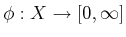. The potential function can be used to define a feedback plan through the use of a local operator, which is a function that selects the action that reduces the potential as much as possible. First, consider the case of a feasible planning problem. The potential function,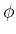, defines a feedback plan by selectingthrough the local operator,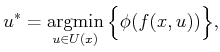(8.4)

which means that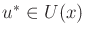is chosen to reduceas much as possible. The local operator yields a kind of greedy descent of the potential. Note that the action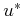may not be unique. In the continuous-space analog to this, the corresponding local operator performs a descent along the negative gradient (often referred to as gradient descent).

In the case of optimal planning, the local operator is defined as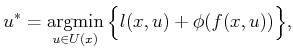(8.5)

which looks similar to the dynamic programming condition, (2.19). It becomes identical to (2.19) ifis interpreted as the optimal cost-to-go. A simplification of (8.5) can be made if the planning problem is isotropic, which means that the cost is the same in every direction: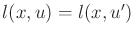for all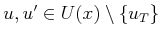. In this case, the cost term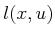does not affect the minimization in (8.5). A common example in which this assumption applies is if the cost functional counts the number of stages required to reach the goal. The costs of particular actions chosen along the way are not important. Using the isotropic property, (8.5) simplifies back to (8.4).

When is a potential function useful? Many useless potential functions can be defined that fail to reach the goal, or cause states to cycle indefinitely, and so on. The most desirable potential function is one that for any initial state causes arrival in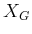, if it is reachable. This requires only a few simple properties. A potential function that satisfies these will be called a navigation function.8.2

Suppose that the cost functional is isotropic. Let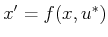, which is the state reached after applying the actionthat was selected by (8.4). A potential function,, is called a (feasible) navigation function if

1.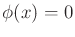for all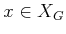.
2.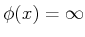if and only if no point inis reachable from.
3. For every reachable state,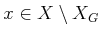, the local operator produces a state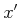for which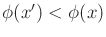.
The first condition requires the goal to have zero potential (this condition is actually not necessary but is included for convenience). The second condition requires that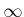serves as a special indicator that the goal is not reachable from some state. The third condition means that the potential function has no local minima except at the goal. This means that the execution of the resulting feedback plan will progress without cycling and the goal region will eventually be reached.

An optimal navigation function is defined as the optimal cost-to-go,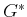. This means that in addition to the three properties above, the navigation function must also satisfy the principle of optimality: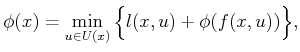(8.6)

which is just (2.18) withreplaced by. See Section 15.2.1 for more on this connection.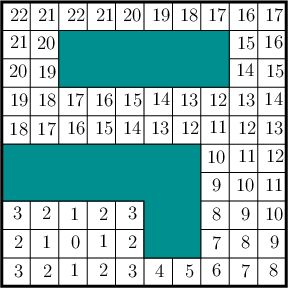Example 8..2 (Navigation Function on a 2D Grid)   Return to the planning problem in Example 8.1. Assume that an isotropic cost model is used: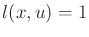if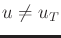. Figure 8.3 shows a navigation function. The numbers shown in the tiles represent. Verify thatsatisfies the three requirements for a navigation function.

At any state, an action is applied that reduces the potential value. This corresponds to selecting the action using (8.4). The process may be repeated from any state untilis reached. This example clearly illustrates how a navigation function can be used as an alternative definition of a feedback plan.Example 8..3 (Airport Terminal)   You may have found yourself using a navigation function to find the exit after arriving in an unfamiliar airport terminal. Many terminals are tree-structured, with increasing gate numbers as the distance to the terminal exit increases. If you wish to leave the terminal, you should normally walk toward the lower numbered gates.Steven M LaValle 2020-08-14